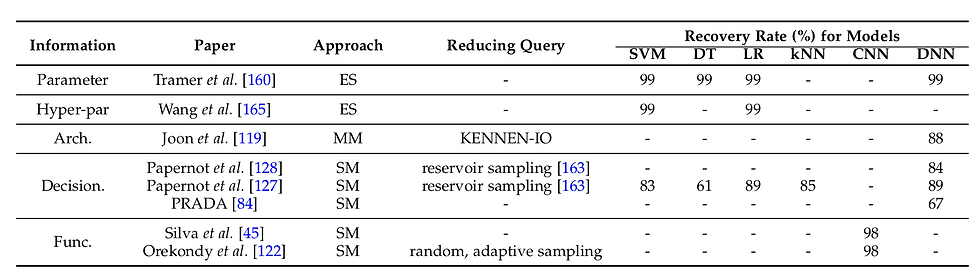top of pageSearch
• Paul Irolla

# What is model stealing and why it matters

Training a model is costly: collecting a mass of relevant samples, preprocessing data to solve a specific problem, finding an effective machine learning model and providing it with the necessary computing power ... What if a competitor could steal this model, which you have just designed, without any particular effort.Overview of a model stealing / model extraction approach 

This attack is known as model stealing attack or model extraction attack. As many adversary attacks in the field, it works by querying the target model with samples and using the model responses to forge a replicated model. Done in a black-box set up, it can serve different purposes:

* Copy an effective model at low cost for its functionnality.

* Copy the model to facilitate the design of other attacks (adversarial samples, membership inference, adversarial reprogramming etc.) with a white-box set up.

Before going into the technique detailing the different ways to steal a model, first I would like to make the following disturbing observation: it is an attack that works really well, and the models can be stolen with a frightful recovery rate.State-of-the-art of model stealing, showing near-perfect recovery rate of stolen models 

## Diving into the technique

Any kind of machine learning (ML) model can be stolen . What is valuable in a model is its functionnality that can recovered by stealing its trained parameters (weights w) or its decision boundaries. The model can be represented as an equation y = f(x, w), with x an input and y an output. By presenting lots of samples to the target model and storing its responses, it is possible to gather enough equations to build an equation system that is solvable, where w are the unknown variables to find. This is very effective on all kind of models given that we know the dimension of w and the model architecture f (i.e. the relation between the input x, the weights w and the output y). Hence this attack works best on a grey-box set up, when we have some information about the model.

In the case where we have no information, we can use a substitute model (a.k.a. a shadow model), meaning a deep learning model that we train to learn the relation between the inputs we present to the target model and its responses. With enough inputs, our shadow model learns the decision boundaries of the target model, actually reproducing its functionnalities. With an access to the confidence levels about each output classes (in a classification task), we can reduce the number of samples presented to the target model, but ultimately output labels only are enough to train the shadow model.

A question naturally arises: what kind of sample should we present at the input of the target model? The best samples are naturally samples similar to those that were used to train the target model. As part of an image recognition problem, we then use data augmentation techniques (transformation in the image space) in order to reduce the number of queries to the model. The case may arise where it is really difficult to obtain samples resembling or of the same category as the original ones. Study  shows that you can steal a model with any type of input, even if there is no relation to the original problem. The authors manage to steal different models for Facial Expression Recognition, General Object Classification and Satellite Crosswalk Classification with roughly the same recovery rate using related images than using ~50x time more images that are unrelated to the problem the target model is trying to solve.

## What can we do about it

If the model is used through a public API, it is unfortunately not possible to completely prevent model theft. What we can do is making the attack more expensive to implement. The best strategy is a strict access control to the model with a limit of daily requests. To this, add a financial cost to the request and give only the output labels and not the confidence levels. If it is necessary to disclose these confidence levels, then you can add a Gaussian noise to the levels to disrupt the theft.

## References

 Tramèr, F., Zhang, F., Juels, A., Reiter, M.K., & Ristenpart, T. (2016). Stealing Machine Learning Models via Prediction APIs. ArXiv, abs/1609.02943.

 He, Y., Meng, G., Chen, K., Hu, X., & He, J. (2019). Towards Privacy and Security of Deep Learning Systems: A Survey. arXiv preprint arXiv:1911.12562.

 J. R. C. da Silva, R. F. Berriel, C. Badue, A. F. de Souza, and T. Oliveira-Santos. Copycat CNN: stealing knowledge by persuading confession with random non-labeled data. In International Joint Conference on Neural Networks, IJCNN, Rio de Janeiro,

Brazil, pages 1–8, July 8-13, 2018.

## Notes

SVM: Support Vector Machine

DT: Decision Tree

LR: Linear regression

kNN: k-nearest neighbours

CNN: Convolutional Neural Network

DNN: Deep Neural Network

ML: Machine Learning

ES: Equation solving

MM: Meta model

SM: Substitute model